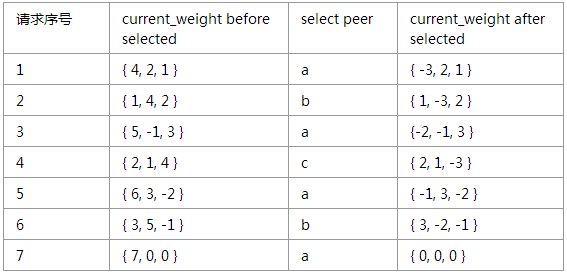# 负载均衡之加权轮询算法

在介绍加权轮询算法(WeightedRound-Robin)之前，首先介绍一下轮询算法(Round-Robin)。

轮询算法是最简单的一种负载均衡算法。它的原理是把来自用户的请求轮流分配给内部的服务器：从服务器1开始，直到服务器N，然后重新开始循环。

算法的优点是其简洁性，它无需记录当前所有连接的状态，所以它是一种无状态调度。

假设有N台服务器：S = {S1, S2, …, Sn}，一个指示变量i表示上一次选择的服务器ID。变量i被初始化为N-1。该算法的伪代码如下：

j = i;
do
{
j = (j + 1) mod n;
i = j;
return Si;
} while (j != i);
return NULL;


轮询算法假设所有服务器的处理性能都相同，不关心每台服务器的当前连接数和响应速度。当请求服务间隔时间变化比较大时，轮询算法容易导致服务器间的负载不平衡。所以此种均衡算法适合于服务器组中的所有服务器都有相同的软硬件配置并且平均服务请求相对均衡的情况。

轮询算法并没有考虑每台服务器的处理能力，实际中可能并不是这种情况。由于每台服务器的配置、安装的业务应用等不同，其处理能力会不一样。所以，加权轮询算法的原理就是：根据服务器的不同处理能力，给每个服务器分配不同的权值，使其能够接受相应权值数的服务请求。

首先看一个简单的Nginx负载均衡配置。

http {
upstream cluster {
server a weight=1;
server b weight=2;
server c weight=4;
}
...
} 

按照上述配置，Nginx每收到7个客户端的请求，会把其中的1个转发给后端a，把其中的2个转发给后端b，把其中的4个转发给后端c。

加权轮询算法的结果，就是要生成一个服务器序列。每当有请求到来时，就依次从该序列中取出下一个服务器用于处理该请求。比如针对上面的例子，加权轮询算法会生成序列{c, c, b, c, a, b, c}。这样，每收到7个客户端的请求，会把其中的1个转发给后端a，把其中的2个转发给后端b，把其中的4个转发给后端c。收到的第8个请求，重新从该序列的头部开始轮询。

总之，加权轮询算法要生成一个服务器序列，该序列中包含n个服务器。n是所有服务器的权重之和。在该序列中，每个服务器的出现的次数，等于其权重值。并且，生成的序列中，服务器的分布应该尽可能的均匀。比如序列{a, a, a, a, a, b, c}中，前五个请求都会分配给服务器a，这就是一种不均匀的分配方法，更好的序列应该是：{a, a, b, a, c, a, a}。

下面介绍两种加权轮询算法：

1：普通加权轮询算法

这种算法的原理是：在服务器数组S中，首先计算所有服务器权重的最大值max(S)，以及所有服务器权重的最大公约数gcd(S)。

index表示本次请求到来时，选择的服务器的索引，初始值为-1；current_weight表示当前调度的权值，初始值为max(S)。

当请求到来时，从index+1开始轮询服务器数组S，找到其中权重大于current_weight的第一个服务器，用于处理该请求。记录其索引到结果序列中。

在轮询服务器数组时，如果到达了数组末尾，则重新从头开始搜索，并且减小current_weight的值：current_weight -= gcd(S)。如果current_weight等于0，则将其重置为max(S)。

该算法的实现代码如下：

#include <stdlib.h>
#include <stdio.h>
#include <unistd.h>
#include <string.h>

typedef struct
{
int weight;
char name;
}server;

int getsum(int *set, int size)
{
int i = 0;
int res = 0;

for (i = 0; i < size; i++)
res += set[i];

return res;
}

int gcd(int a, int b)
{
int c;
while(b)
{
c = b;
b = a % b;
a = c;
}

return a;
}

int getgcd(int *set, int size)
{
int i = 0;
int res = set;

for (i = 1; i < size; i++)
res = gcd(res, set[i]);

return res;
}

int getmax(int *set, int size)
{
int i = 0;
int res = set;

for (i = 1; i < size; i++)
{
if (res < set[i]) res = set[i];
}

return res;
}

int lb_wrr__getwrr(server *ss, int size, int gcd, int maxweight, int *i, int *cw)
{
while (1)
{
*i = (*i + 1) % size;
if (*i == 0)
{
*cw = *cw - gcd;
if (*cw <= 0)
{
*cw = maxweight;
if (*cw == 0)
{
return -1;
}
}
}
if (ss[*i].weight >= *cw)
{
return *i;
}
}
}

void wrr(server *ss, int *weights, int size)
{
int i = 0;

int gcd = getgcd(weights, size);
int max = getmax(weights, size);
int sum = getsum(weights, size);

int index = -1;
int curweight = 0;

for (i = 0; i < sum; i++)
{
lb_wrr__getwrr(ss, size, gcd, max, &(index), &(curweight));
printf("%s(%d) ", ss[index].name, ss[index].weight);
}

printf("\n");
return;
}

server *initServers(char **names, int *weights, int size)
{
int i = 0;
server *ss = calloc(size, sizeof(server));

for (i = 0; i < size; i++)
{
ss[i].weight = weights[i];
memcpy(ss[i].name, names[i], 2);
}

return ss;
}

int main()
{
int i = 0;

int weights[] = {1, 2, 4};
char *names[] = {"a", "b", "c"};
int size = sizeof(weights) / sizeof(int);

server *ss = initServers(names, weights, size);

printf("server is ");
for (i = 0; i < size; i++)
{
printf("%s(%d) ", ss[i].name, ss[i].weight);
}
printf("\n");

printf("\nwrr sequence is ");
wrr(ss, weights, size);

return;
}

上面的代码中，算法的核心部分就是wrr和lb_wrr__getwrr函数。在wrr函数中，首先计算所有服务器权重的最大公约数gcd，权重最大值max，以及权重之和sum。

初始时，index为-1，curweight为0，然后依次调用lb_wrr__getwrr函数，得到本次选择的服务器索引index。

算法的核心思想体现在lb_wrr__getwrr函数中。以例子说明更好理解一些：对于服务器数组{a(1), b(2), c(4)}而言，gcd为1，maxweight为4。

第1次调用该函数时，i(index)为-1，cw(current_weight)为0，进入循环后，i首先被置为0，因此cw被置为maxweight。从i开始轮询服务器数组ss，第一个权重大于等于cw的服务器是c，因此，i被置为2，并返回其值。

第2次调用该函数时，i为2，cw为maxweight。进入循环后，i首先被置为0，因此cw被置为cw-gcd，也就是3。从i开始轮询服务器数组ss，第一个权重大于等于cw的服务器还是c，因此，i被置为2，并返回其值。

第3次调用该函数时，i为2，cw为3。进入循环后，i首先被置为0，因此cw被置为cw-gcd，也就是2。从i开始轮询服务器数组ss，第一个权重大于等于cw的服务器是b，因此，i被置为1，并返回其值。

第4次调用该函数时，i为1，cw为2。进入循环后，i首先被置为2，从i开始轮询服务器数组ss，第一个权重大于等于cw的服务器是c，因此，i被置为2，并返回其值。

第5次调用该函数时，i为2，cw为2。进入循环后，i首先被置为0，因此cw被置为cw-gcd，也就是1。从i开始轮询服务器数组ss，第一个权重大于等于cw的服务器是a，因此，i被置为0，并返回其值。

第6次调用该函数时，i为0，cw为1。进入循环后，i首先被置为1，从i开始轮询服务器数组ss，第一个权重大于等于cw的服务器是b，因此，i被置为1，并返回其值。

第7次调用该函数时，i为1，cw为1。进入循环后，i首先被置为2，从i开始轮询服务器数组ss，第一个权重大于等于cw的服务器是c，因此，i被置为2，并返回其值。

经过7（1+2+4）次调用之后，每个服务器被选中的次数正好是其权重值。上面程序的运行结果如下：

server is a(1) b(2) c(4)

wrr sequence is c(4) c(4) b(2) c(4) a(1) b(2) c(4)


如果有新的请求到来，第8次调用该函数时，i为2，cw为1。进入循环后，i首先被置为0，cw被置为cw-gcd，也就是0，因此cw被重置为maxweight。这种情况就跟第一次调用该函数时一样了。因此，7次是一个轮回，7次之后，重复之前的过程。

这背后的数学原理，自己思考了一下，总结如下：

current_weight的值，其变化序列就是一个等差序列：max, max-gcd, max-2gcd, …, 0(max)，将current_weight从max变为0的过程，称为一个轮回。

针对每个current_weight，该算法就是要把服务器数组从头到尾扫描一遍，将其中权重大于等于current_weight的所有服务器填充到结果序列中。扫描完一遍服务器数组之后，将current_weight变为下一个值，再一次从头到尾扫描服务器数组。

在current_weight变化过程中，不管current_weight当前为何值，具有max权重的服务器每次肯定会被选中。因此，具有max权重的服务器会在序列中出现max/gcd次（等差序列中的项数）。

更一般的，当current_weight变为x之后，权重为x的服务器，在current_weight接下来的变化过程中，每次都会被选中，因此，具有x权重的服务器，会在序列中出现x/gcd次。所以，每个服务器在结果序列中出现的次数，是与其权重成正比的，这就是符合加权轮询算法的要求了。

2：平滑的加权轮询

上面的加权轮询算法有个缺陷，就是某些情况下生成的序列是不均匀的。比如针对这样的配置：

http {
upstream cluster {
server a weight=5;
server b weight=1;
server c weight=1;
}
...
} 

生成的序列是这样的：{a,a, a, a, a, c, b}。会有5个连续的请求落在后端a上，分布不太均匀。

在Nginx源码中，实现了一种叫做平滑的加权轮询（smooth weighted round-robin balancing）的算法，它生成的序列更加均匀。比如前面的例子，它生成的序列为{ a, a, b, a, c, a, a}，转发给后端a的5个请求现在分散开来，不再是连续的。

该算法的原理如下：

每个服务器都有两个权重变量：

a：weight，配置文件中指定的该服务器的权重，这个值是固定不变的；

b：current_weight，服务器目前的权重。一开始为0，之后会动态调整。

每次当请求到来，选取服务器时，会遍历数组中所有服务器。对于每个服务器，让它的current_weight增加它的weight；同时累加所有服务器的weight，并保存为total。

遍历完所有服务器之后，如果该服务器的current_weight是最大的，就选择这个服务器处理本次请求。最后把该服务器的current_weight减去total。

上述描述可能不太直观，来看个例子。比如针对这样的配置：

http {
upstream cluster {
server a weight=4;
server b weight=2;
server c weight=1;
}
...
} 

按照这个配置，每7个客户端请求中，a会被选中4次、b会被选中2次、c会被选中1次，且分布平滑。我们来算算看是不是这样子的。

initial  current_weight  of a, b, c is {0, 0, 0}通过上述过程，可得以下结论：

a：7个请求中，a、b、c分别被选取了4、2、1次，符合它们的权重值。

b：7个请求中，a、b、c被选取的顺序为a, b,a, c, a, b, a，分布均匀，权重大的后端a没有被连续选取。

c：每经过7个请求后，a、b、c的current_weight又回到初始值{0, 0,0}，因此上述流程是不断循环的。

根据该算法实现的代码如下：

#include <stdlib.h>
#include <stdio.h>
#include <unistd.h>
#include <string.h>

typedef struct
{
int weight;
int cur_weight;
char name;
}server;

int getsum(int *set, int size)
{
int i = 0;
int res = 0;

for (i = 0; i < size; i++)
res += set[i];

return res;
}

server *initServers(char **names, int *weights, int size)
{
int i = 0;
server *ss = calloc(size+1, sizeof(server));

for (i = 0; i < size; i++)
{
ss[i].weight = weights[i];
memcpy(ss[i].name, names[i], 3);
ss[i].cur_weight = 0;
}
return ss;
}

int getNextServerIndex(server *ss, int size)
{
int i ;
int index = -1;
int total = 0;

for (i = 0; i < size; i++)
{
ss[i].cur_weight += ss[i].weight;
total += ss[i].weight;

if (index == -1 || ss[index].cur_weight < ss[i].cur_weight)
{
index = i;
}
}

ss[index].cur_weight -= total;
return index;
}

void wrr_nginx(server *ss, int *weights, int size)
{
int i = 0;
int index = -1;
int sum = getsum(weights, size);

for (i = 0; i < sum; i++)
{
index = getNextServerIndex(ss, size);
printf("%s(%d) ", ss[index].name, ss[index].weight);
}
printf("\n");
}

int main()
{
int i = 0;
int weights[] = {4, 2, 1};
char *names[] = {"a", "b", "c"};
int size = sizeof(weights) / sizeof(int);

server *ss = initServers(names, weights, size);

printf("server is ");
for (i = 0; i < size; i++)
{
printf("%s(%d) ", ss[i].name, ss[i].weight);
}
printf("\n");

printf("\nwrr_nginx sequence is ");
wrr_nginx(ss, weights, size);

return;
}


上述代码的运行结果如下：

server is a(4) b(2) c(1)

wrr_nginx sequence is a(4) b(2) a(4) c(1) a(4) b(2) a(4)


如果服务器配置为：{a(5),b(1), c(1)}，则运行结果如下：

server is a(5) b(1) c(1)

wrr_nginx sequence is a(5) a(5) b(1) a(5) c(1) a(5) a(5)


可见，该算法生成的序列确实更加均匀。

该算法背后的数学原理，实在没想出来，google也没查到相关论证……，等待后续查证了。

负载均衡算法，一般要伴随健康检查算法一起使用。健康检查算法的作用就是对所有的服务器进行存活和健康检测，看是否需要提供给负载均衡做选择。如果一台机器的服务出现了问题，健康检查就会将这台机器从服务列表中去掉，让负载均衡算法看不到这台机器的存在。

具体在加权轮询算法中，当健康检查算法检测出某服务器的状态发生了变化，比如从UP到DOWN，或者反之时，就会更新权重，重新计算结果序列。

http://kb.linuxvirtualserver.org/wiki/Weighted_Round-Robin_Scheduling

http://ialloc.org/posts/2014/11/14/ngx-notes-module-http-sticky/

http://blog.csdn.net/zhangskd/article/details/50194069